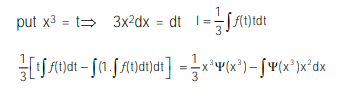# Solve the equation

Question:

If $\int f(\mathrm{x}) \mathrm{dx}=\Psi(\mathrm{x})$, then $\int \mathrm{x}^{5} f\left(\mathrm{x}^{3}\right) \mathrm{dx}$ is equal to :

1. $\frac{1}{3}\left[x^{3} \Psi\left(x^{3}\right)-\int x^{2} \Psi\left(x^{3}\right) d x\right]+C$

2. $\frac{1}{3} \mathrm{x}^{3} \Psi\left(\mathrm{x}^{3}\right)-3 \int \mathrm{x}^{3} \Psi\left(\mathrm{x}^{3}\right) \mathrm{dx}+\mathrm{C}$

3. $\frac{1}{3} x^{3} \Psi\left(x^{3}\right)-\int x^{2} \Psi\left(x^{3}\right) d x+C$

4. $\frac{1}{3}\left[x^{3} \Psi\left(x^{3}\right)-\int x^{3} \Psi\left(x^{3}\right) d x\right]+C$

Correct Option: , 3

Solution: